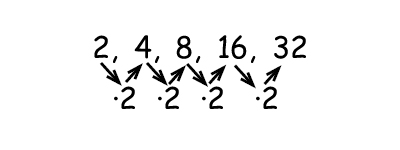# Geometric sequences of numbers

A geometric sequence increase or decrease by a common factor - the common ratio.

ExampleThe common ratio is 2. We can write this as an algebraic expression.

$a_{n}=a_{1}\cdot r^{n-1}$

Where an is the first number (in this case 2).

r is the common factor (in this case 2).

n is the place in the set the number has (2 has n = 1, 8 has n = 3 etc.

We can rewrite this formula for our specific sequence:

$a_{n}=2\cdot 2^{n-1}$

If we would like to know the next number in the sequence after 32. The next number in the sequence is the 6th number. This gives us:

Example

$a_{n}=a\cdot r^{n-1}$

$a_{6}=2\cdot 2^{6-1}$

$a_{6}=2\cdot 2^{5}$

$a_{6}=2\cdot 32$

$a_{6}=64$

## Video lesson

Find the eighth number